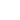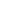+36-20-577-9020info@bushungary.com

# Booking

Calculated price: 75 €

Calculated price: 80 €

Calculated price: 90 €

Calculated price: 100 €

Calculated price: 110 €

Calculated price: 140 €

Calculated price: 150 €

Calculated price: 170 €

Calculated price: 190 €

Calculated price: 200 €